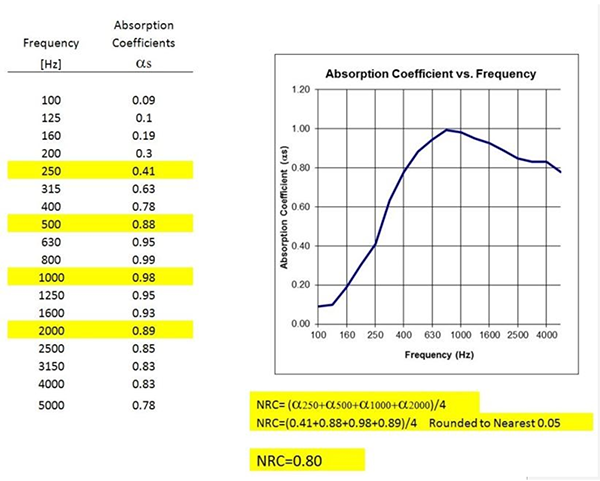# How Materials Absorb Sound

August 9, 2022   -

## EXAMINING HOW MATERIALS ABSORB SOUND: Noise Reduction Coefficient

When sound interacts with any material, it can be either:

• Reflected
• Transmitted
• Absorbed

The measure of how well a material absorbs sound is called the "Noise Reduction Coefficient" or NRC. ASTM defines NRC as a single-number rating, the average, rounded to the nearest 0.05, of the sound absorption coefficients of material for the four 1/3-octave test frequency bands at 250, 500, 100, and 2000 Hz. The absorption coefficients are measured according to ASTM Test Method C423. This entails measuring the time required for the sound field to decay 60 dB (decibels) in a Reverberation Room. Using the Sabine Equation, the absorption of the Room — both with and without a 72-square-foot test sample — can be calculated. The difference in the absorptions between the Sample Phase and Empty Room Phase is the absorption of the sample per square foot. This is called the Absorption Coefficient.

The Sabine Equation proves there is a relationship between the quality of acoustics, the size of the chamber, and the amount of absorption surface present. Reverberation time is the most important characteristic to gauge the acoustical quality of a room.

The unit of measured absorption is the sabin, named in honor of Wallace Sabine (1868-1919). He developed the Sabine Equation, and is considered "the father of architectural acoustics." An American physicist, Sabine graduated from Ohio State University at age 18, completing his graduate studies at Harvard University and then continuing his tenure there as a faculty member.